Boynton and Bramley equation of state

The Boynton and Bramley equation of state is given by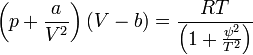$\left( p + \frac{a}{V^2}\right) (V-b) = \frac{RT}{\left(1+ \frac{\psi^2}{T^2}\right)}$

where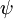$\psi$ is a characteristic temperature. and where:

•$p$ is the pressure,
•$V$ is the volume,
•$T$ is the absolute temperature,
•$R$ is the molar gas constant;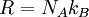$R = N_A k_B$, with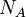$N_A$ being the Avogadro constant and$k_B$ being the Boltzmann constant.
•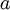$a$ and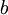$b$ are constants that introduce the effects of attraction and volume respectively and depend on the substance in question.

For this equation at the critical point one has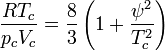$\frac{RT_c}{p_cV_c} = \frac{8}{3}\left( 1 + \frac{\psi^2}{T_c^2}\right)$